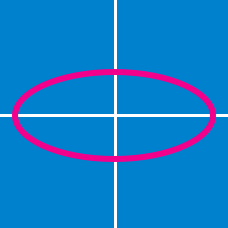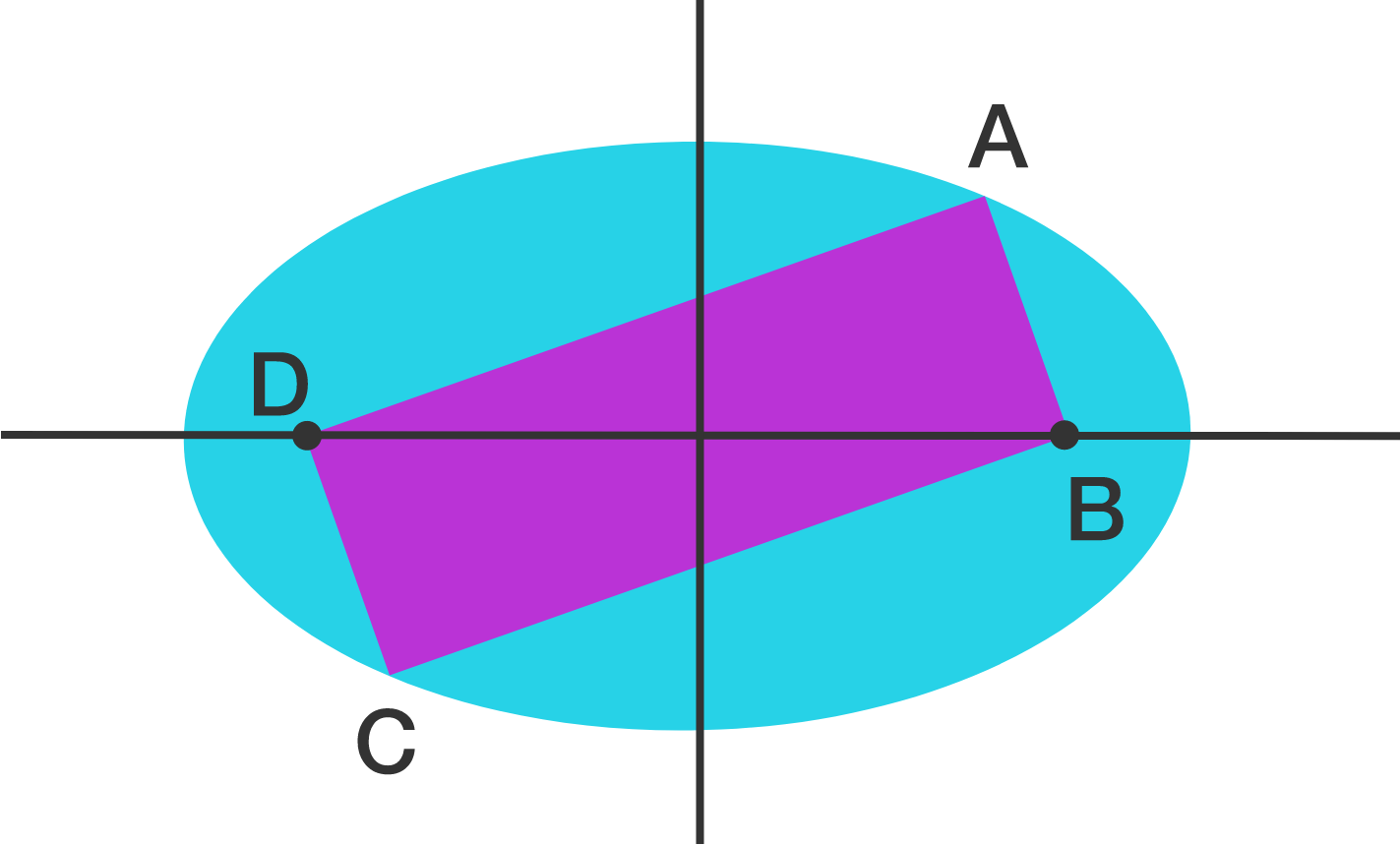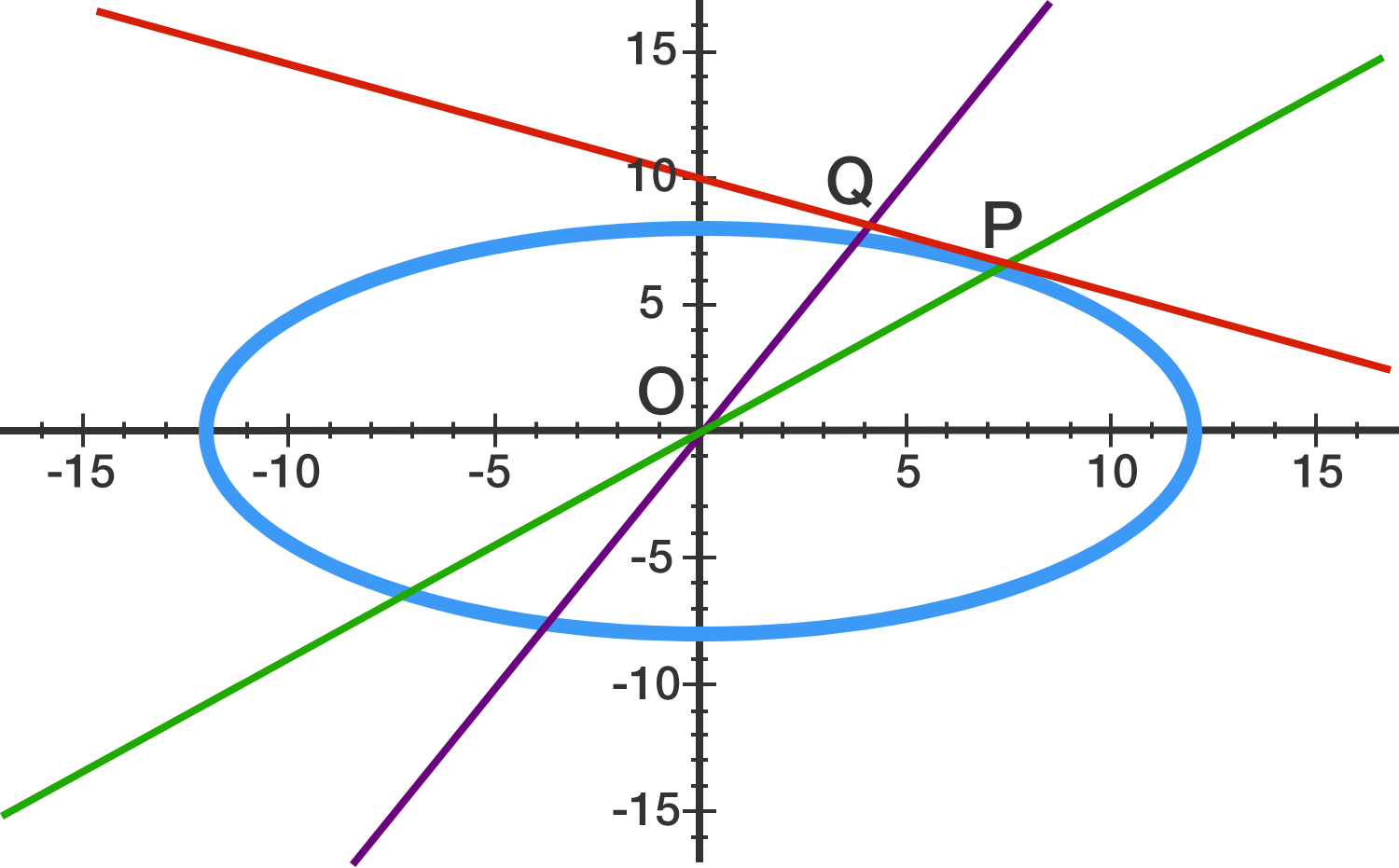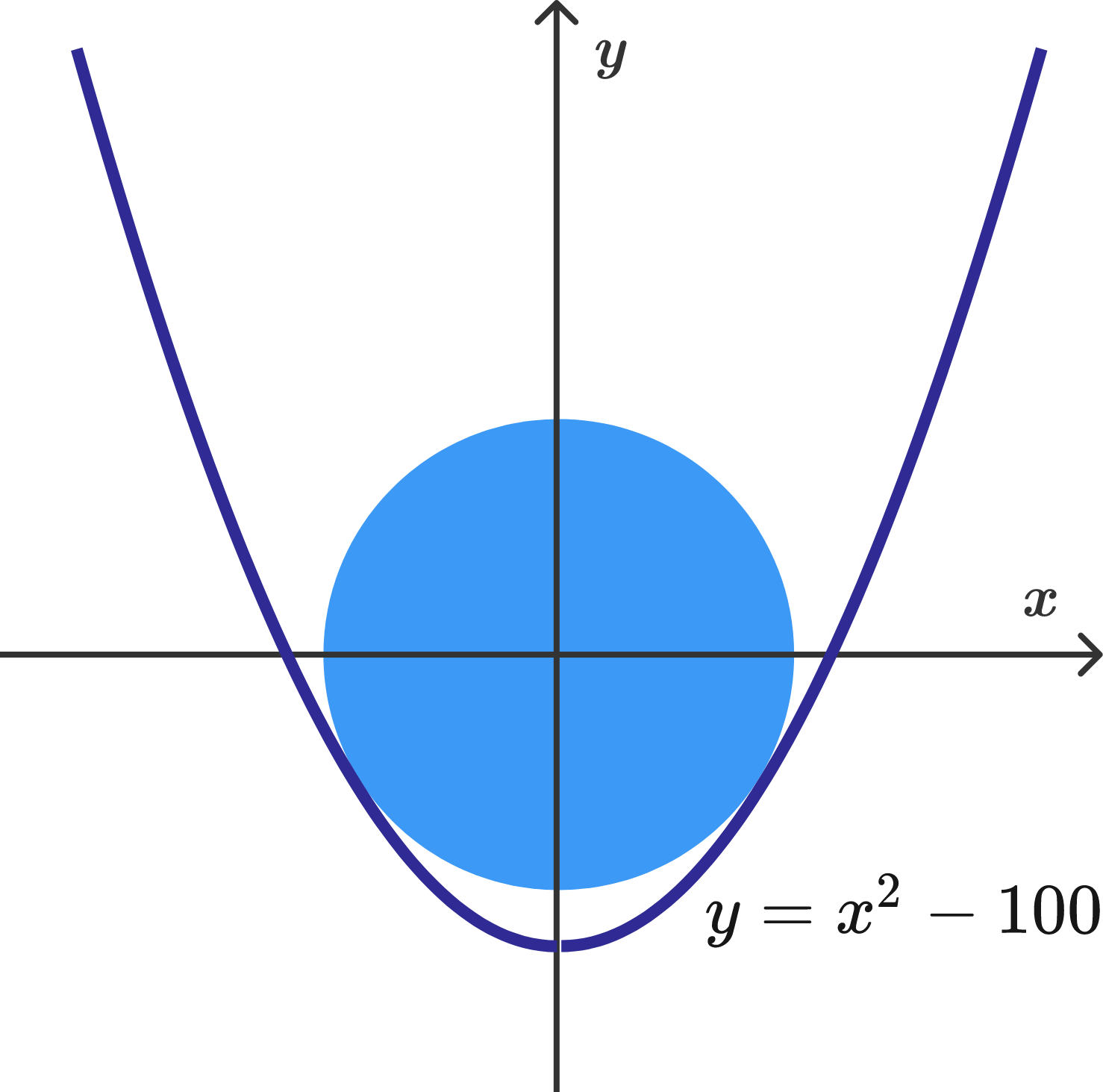Geometry

Conic Sections: Level 3 ChallengesThe figure above shows two ellipses whose major axes are perpendicular to each other. Each ellipse passes through the other ellipse’s foci, which form the vertices of a square. If the shaded square encloses an area of 16, then what is the area enclosed by one of the ellipses?

Find the shortest distance between the parabolas $2y^2=2x-1$ and $2x^2=2y-1$.$A,B,C,D$ are consecutive vertices of a rectangle whose area is $2006$ square units. An ellipse with area $2006\pi$, which passes through $A$ and $C$ has its foci at $B$ and $D$.

If the perimeter of the rectangle can be expressed as $p\sqrt{q}$ where $p$ is a positive integer and $q$ is a square-free positive integer, find $p+q$.Consider an ellipse $\dfrac{x^2}{144} + \dfrac{y^2}{64} = 1$. A line is drawn tangent to the ellipse at a point $P$. A line segment drawn from the origin to a point $Q$ on this line is perpendicular to this tangent line.

Find the maximum area of $\triangle POQ$.

The area of a circle centered at the origin, which is inscribed in the parabola $y=x^2-100,$ can be expressed as $\frac{a}{b} \pi,$ where $a$ and $b$ are coprime positive integers. What is the value of $a+b$?×